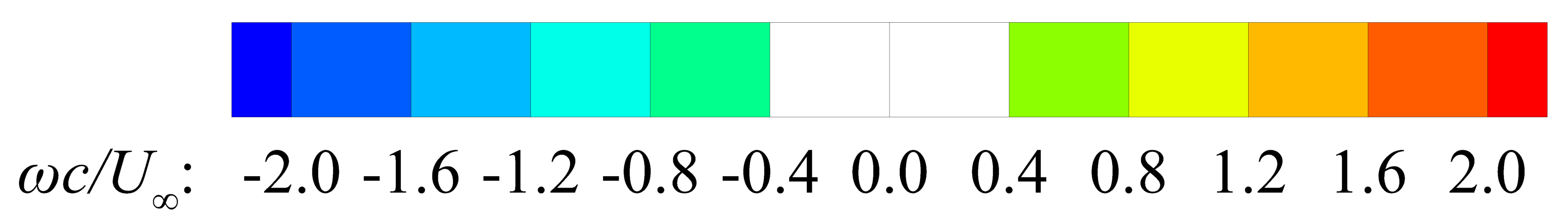## Movies

B. Zhang and C. Liang, "High-Order Numerical Simulation of Flapping Wing for Energy Harvesting", AIAA Paper, 2019-3338, June, 2019. doi:10.2514/6.2019-3338

1. Flow field when heaving leads pitching with a phase angle $\phi=0^\circ$
2. Flow field when heaving leads pitching with a phase angle $\phi=30^\circ$
3. Flow field when heaving leads pitching with a phase angle $\phi=60^\circ$
4. Flow field when heaving leads pitching with a phase angle $\phi=90^\circ$
5. Flow field when heaving leads pitching with a phase angle $\phi=120^\circ$
6. Flow field when heaving leads pitching with a phase angle $\phi=150^\circ$
7. Flow field when heaving leads pitching with a phase angle $\phi=180^\circ$TOP